BE-proff
●

2015-08-13 13:44
(2560 d 01:05 ago)

Posting: # 15255
Views: 11,929

## Power calculation [🇷 for BE/BA]

Dear All,

Can anybody share R-code for power calculation using?• CV,
• alpha-error,
• upper&lower limits,
• true means ratio,
• sample size.
Helmut
★★★Vienna, Austria,
2015-08-13 13:59
(2560 d 00:51 ago)

@ BE-proff
Posting: # 15256
Views: 10,875

## Power calculation

Hi BE-proff,

do you mean sumfink which is not covered by PowerTOST’s functions
• power.dp()
• power.noninf()
• power.NTIDFDA()
• power.RatioF()
• power.RSABE()
• power.scABEL()
• power.TOST()
or do you want to re-invent the wheel?

In your list some parameters are missing which are required:
• The statistical method (TOST, reference-scaling, non-inferiority, levels of dose-proportinality studies, whatsoever).
• The design (parallel, crossover, replicate).

Dif-tor heh smusma 🖖Helmut SchützThe quality of responses received is directly proportional to the quality of the question asked. 🚮
Science Quotes
BE-proff
●

2015-08-13 14:44
(2560 d 00:06 ago)

@ Helmut
Posting: # 15257
Views: 10,843

## Power calculation

Hi Helmut,

We say - to re-invent bicycleI will accept all available scripts (if possible) but for BE studies I need cross-over, parallel, replicative designs (judging on PASS).

As for statistic methods - sorry but I am not strong in them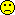Helmut
★★★Vienna, Austria,
2015-08-13 14:58
(2559 d 23:52 ago)

@ BE-proff
Posting: # 15258
Views: 10,965

## Example(s)

Hi BE-proff,

» I will accept all available scripts (if possible) but for BE studies I need cross-over, parallel, replicative designs (judging on PASS).

After library(PowerTOST) type help(package=PowerTOST). All linked functions contain not only de­scrip­tions of required parameters (and their defaults – which you could leave out) but at the end some examples. Compare them to PASS (or the other way ’round).

Edit: Code to explore this example.

library(PowerTOST) N     <- c(50, 150, 250, 350, 450, 550) # vector of sample sizes RL    <- 0.9                            # lower acceptance limit UL    <- 1/RL                           # upper acceptance limit R1    <- 1                              # assumed ratio COV   <- 0.5                            # assumed CV Alpha <- 0.05                           # guess what! Ns    <- length(N)                      # number of sample sizes                                         # to explore res   <- matrix(nrow=Ns, ncol=8, byrow=T,            dimnames=list(NULL,            c("Power", "N", "RL", "RU", "R1", "COV", "Alpha", "Beta")))                                         # Define the result matrix:                                         # 6 rows (sample size)                                         # 8 columns                                         # vector of column headers res[, 2] <- N                           # fill in the given stuff res[, 3] <- rep(RL, Ns)                 # to the respective res[, 4] <- rep(UL, Ns)                 # cells of the res[, 5] <- rep(R1, Ns)                 # matrix res[, 6] <- rep(COV, Ns) res[, 7] <- rep(Alpha, Ns) for(j in 1:Ns) {                        # loop over sample sizes   res[j, 1] <- power.TOST(alpha=Alpha, CV=COV, theta0=R1,                  theta1=RL, theta2=UL, n=N[j], method="exact") } res[, 8] <- 1-res[, 1] res <- data.frame(round(res, 4))        # cosmetics for print(res, row.names=F)                 # printing

Gives…

  Power   N  RL     RU R1 COV Alpha   Beta  0.0000  50 0.9 1.1111  1 0.5  0.05 1.0000  0.2190 150 0.9 1.1111  1 0.5  0.05 0.7810  0.6002 250 0.9 1.1111  1 0.5  0.05 0.3998  0.8064 350 0.9 1.1111  1 0.5  0.05 0.1936  0.9101 450 0.9 1.1111  1 0.5  0.05 0.0899  0.9596 550 0.9 1.1111  1 0.5  0.05 0.0404

… which exactly match PASS’ results. I was wrong (and Detlew right). Here PASS’ uses the exact method.

For the noncentral t I got…

  Power   N  RL     RU R1 COV Alpha   Beta  0.0000  50 0.9 1.1111  1 0.5  0.05 1.0000  0.2189 150 0.9 1.1111  1 0.5  0.05 0.7811  0.6002 250 0.9 1.1111  1 0.5  0.05 0.3998  0.8064 350 0.9 1.1111  1 0.5  0.05 0.1936  0.9101 450 0.9 1.1111  1 0.5  0.05 0.0899  0.9596 550 0.9 1.1111  1 0.5  0.05 0.0404

Slight differences for low samples sizes compared to the exact method.

Occasionally slight differences for the parallel design example.

library(PowerTOST) N1    <- seq(50, 550, 100) N2    <- N1 N     <- N1+N2 RL    <- 0.8 UL    <- 1/RL R1    <- c(1, 1.05) COV   <- 1.5 Alpha <- 0.05 Ns    <- length(N) Rs    <- length(R1) res   <- matrix(nrow=Ns*Rs, ncol=9, byrow=T,            dimnames=list(NULL,            c("Power", "N1", "N2", "N", "R1", "COV", "RL", "RU", "Alpha"))) res[, 2] <- rep(N1, Rs) res[, 3] <- rep(N2, Rs) res[, 4] <- rep(N, Rs) res[, 5] <- rep(R1, each=Ns) res[, 6] <- rep(COV, Ns*Rs) res[, 7] <- rep(RL, Ns*Rs) res[, 8] <- rep(UL, Ns*Rs) res[, 9] <- rep(Alpha, Ns*Rs) l     <- 0 for(k in 1:Rs) {   # loop over ratios   for(j in 1:Ns) { # loop over sample sizes     l <- l+1     res[l, 1] <- power.TOST(alpha=Alpha, CV=COV, theta0=R1[k],                    theta1=RL, theta2=UL, n=N[j], method="exact",                    design="parallel")   } } res <- data.frame(round(res, 5)) print(res, row.names=F)    Power  N1  N2    N   R1 COV  RL   RU Alpha  0.00000  50  50  100 1.00 1.5 0.8 1.25  0.05  0.10488 150 150  300 1.00 1.5 0.8 1.25  0.05  0.48431 250 250  500 1.00 1.5 0.8 1.25  0.05  0.71606 350 350  700 1.00 1.5 0.8 1.25  0.05  0.84896 450 450  900 1.00 1.5 0.8 1.25  0.05  0.92185 550 550 1100 1.00 1.5 0.8 1.25  0.05  0.00000  50  50  100 1.05 1.5 0.8 1.25  0.05  0.09731 150 150  300 1.05 1.5 0.8 1.25  0.05  0.43421 250 250  500 1.05 1.5 0.8 1.25  0.05  0.63561 350 350  700 1.05 1.5 0.8 1.25  0.05  0.75960 450 450  900 1.05 1.5 0.8 1.25  0.05  0.83925 550 550 1100 1.05 1.5 0.8 1.25  0.05

Dif-tor heh smusma 🖖Helmut SchützThe quality of responses received is directly proportional to the quality of the question asked. 🚮
Science Quotes
d_labes
★★★

Berlin, Germany,
2015-08-14 10:29
(2559 d 04:21 ago)

@ Helmut
Posting: # 15263
Views: 10,990

## Power parallel group: PowerTOST/SAS/PASS

Dear Helmut,

» Occasionally slight differences for the parallel design example.

As you might imagine: that makes me (as a nitpicker) a little bit nervous.
But of course I trust in my software even if I myself had written it. I only don't trust in any computers.
Fired up my beasty beast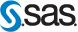.
Used the prudish beautyProc Power;   twosamplemeans   test=equiv_ratio   power=.           /* means we solve for power */   alpha=0.05   meanratio=1 1.05   npergroup=50 150 250 350 450 550   cv=1.5   lower=0.8   upper=1.25   ; run;

Let's look at the results (5 decimals, PASS 14 from your link):
                                             ------ power ------   N1  N2    N   R1 COV  RL   RU Alpha  PowerTOST    SAS    PASS14   PASS12(help) PASS12(calc)   50  50  100 1.00 1.5 0.8 1.25  0.05   0.00000   0.00000  0.00000    0.0000     0.00000  150 150  300 1.00 1.5 0.8 1.25  0.05   0.10488   0.10488  0.10488    0.1049     0.10488  250 250  500 1.00 1.5 0.8 1.25  0.05   0.48431   0.48431  0.48431    0.4843     0.48431  350 350  700 1.00 1.5 0.8 1.25  0.05   0.71606   0.71606  0.71704    0.7170     0.71704  450 450  900 1.00 1.5 0.8 1.25  0.05   0.84896   0.84896  0.84945    0.8494     0.84945  550 550 1100 1.00 1.5 0.8 1.25  0.05   0.92185   0.92185  0.92208    0.9221     0.92208        50  50  100 1.05 1.5 0.8 1.25  0.05   0.00000   0.00000  0.00000    0.0000     0.00000  150 150  300 1.05 1.5 0.8 1.25  0.05   0.09731   0.09731  0.09731    0.1010 (!) 0.09731  250 250  500 1.05 1.5 0.8 1.25  0.05   0.43421   0.43421  0.43421    0.4360 (!) 0.43421  350 350  700 1.05 1.5 0.8 1.25  0.05   0.63561   0.63561  0.63661    0.6366     0.63661  450 450  900 1.05 1.5 0.8 1.25  0.05   0.75960   0.75960  0.76018    0.7602     0.76018  550 550 1100 1.05 1.5 0.8 1.25  0.05   0.83925   0.83925  0.83961    0.8396     0.83961
Note especially the differences between help page and actually calculated for PASS12.

Victory all a long the line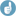! PowerTOST must be correct because SAS says so.

"Enjoy the best sample size software on the market" (PASS12 startup screen).

Power: That which statisticians are always calculating but never have.
Guernsey McPerson's "The Devil's Drug Development Dictionaries"

Regards,

Detlew
Helmut
★★★Vienna, Austria,
2015-08-14 15:34
(2558 d 23:16 ago)

@ d_labes
Posting: # 15269
Views: 10,633

## Power parallel group: PowerTOST/SAS/PASS

Dear Detlew,

» Let's look at the results (5 decimals, PASS 14 from your link): […]
» Note especially the differences between help page and actually calculated for PASS12.

Amazing. At least the algo didn’t change between v12 and 14. Seems that the the guy writing the v12 help page wasn’t particular proficient in copy/pasting. No QA in place?

Dif-tor heh smusma 🖖Helmut SchützThe quality of responses received is directly proportional to the quality of the question asked. 🚮
Science Quotes
d_labes
★★★

Berlin, Germany,
2015-08-14 10:50
(2559 d 03:59 ago)

@ BE-proff
Posting: # 15264
Views: 10,634

## Slippery ground

Dear BE-proff,

» As for statistic methods - sorry but I am not strong in themBeware! Here you are on very slippery ground. Power calculation / sample size estimation are irresolvable connected to the statistical test methods you intend to use in the evaluation of your study results. The test (method) determines how the power hast to be calculated. It determines further the parameters necessary to do power calculations

Without an at least basic understanding of the statistics to use you can't IMHO do any reliable power calculation / sample size estimation. A cook book like approach as requested above doesn't work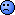.

I highly recommend you the lectures/presentations Helmut has given on many places around the world. Especially those about sample size.
If you need some guidance how to use PowerTOST you may also search this forum. There are many discussions with that topic out there. Have a look f.i. into this post.

Regards,

Detlew
Helmut
★★★Vienna, Austria,
2015-08-14 16:41
(2558 d 22:09 ago)

@ d_labes
Posting: # 15270
Views: 10,931

Dear Detlew and BE-proff,

» Beware! Here you are on very slippery ground.

Full ACK!

» Have a look f.i. into this post.

THX for pointing to this oldie! Below an advanced example. Sometimes both AUC and Cmax are highly variable. According to the EMA we are allowed to widen the limits only for the latter. Which PK-metric will drive the sample size? How large should it be? Which power can we expect for the other metric? How far can T deviate from R whilst still keeping power?

#### input section start #### CV.Cmax  <- 0.55    # HVD/HVDP CV.AUC   <- 0.40    # as well! TR.Cmax  <- 0.90    # suggested for HVDs/HVDPs TR.AUC   <- 0.90    # same! May change to a nicer value if CV <0.3 pwr      <- 0.8     # target #### input section end #### library(PowerTOST) f1       <- function(x) power.scABEL(CV=CV.Cmax, theta0=x,                                      n=n1.AUC, des=des)-pwr f2       <- function(x) power.TOST(CV=CV.AUC, theta0=x,                                    n=n1.Cmax, des=des)-pwr ifelse(CV.Cmax <= 0.5, LL <- exp(-0.76*CV2se(CV.Cmax)),   LL <- exp(-0.76*CV2se(0.5)))    # lower scaled limit if (CV.Cmax <= 0.3) LL <- 0.8     # lower conventional limit res      <- matrix(nrow=2, ncol=7, byrow=T, dimnames=list(NULL,               c("Method", "metric", "GMR", "CV", "n",                 "power", "GMRlo"))) res.Cmax <- sampleN.scABEL(CV=CV.Cmax, theta0=TR.Cmax,               targetpower=pwr, des=des,               details=F, print=F) # scaling allowed n1.Cmax  <- res.Cmax[["Sample size"]] pwr.Cmax <- res.Cmax[["Achieved power"]] res.AUC  <- sampleN.TOST(CV=CV.AUC, theta0=TR.AUC,               targetpower=pwr, des=des,               print=F)            # ABE n1.AUC   <- res.AUC[["Sample size"]] pwr.AUC  <- res.AUC[["Achieved power"]] if(n1.Cmax >= n1.AUC) {   pwr.AUC <- power.TOST(CV=CV.AUC, theta0=TR.AUC, n=n1.Cmax,                         des=des)   TR.AUC.min <- uniroot(f2, interval=c(0.8, TR.AUC), tol=1e-6)$root cat(paste0("PK metric driving the sample size: Cmax\n", "Sample size: ", n1.Cmax, " (ABEL, power: ", sprintf("%.4f", pwr.Cmax), ")\n", "Power of AUC (ABE): ", sprintf("%.4f", pwr.AUC), "\n", "Lowest T/R-ratio of AUC which will give ", pwr, " power: ", sprintf("%.4f", TR.AUC.min), "\n")) res[1, ] <- c("ABEL", "Cmax", sprintf("%.2f", TR.Cmax), sprintf("%.2f", CV.Cmax), n1.Cmax, sprintf("%.4f", pwr.Cmax), NA) res[2, ] <- c("ABE", "AUC", sprintf("%.2f", TR.AUC), sprintf("%.2f", CV.AUC), n1.Cmax, sprintf("%.4f", pwr.AUC), sprintf("%.4f", TR.AUC.min)) } else { pwr.Cmax <- power.scABEL(CV=CV.Cmax, theta0=TR.Cmax, n=n1.AUC, des=des) TR.Cmax.min <- uniroot(f1, interval=c(LL, TR.Cmax), tol=1e-6)$root   cat(paste0("PK metric driving the sample size: AUC\n",     "Sample size: ", n1.AUC, " (ABE, power: ",     sprintf("%.4f", pwr.AUC), ")\n",     "Power of Cmax (ABEL): ", sprintf("%.4f", pwr.Cmax), "\n",     "Lowest T/R-ratio of Cmax which will give ", pwr, " power: ",     sprintf("%.4f", TR.Cmax.min), "\n"))   res[1, ] <- c("ABE", "AUC", sprintf("%.2f", TR.AUC),                  sprintf("%.2f", CV.AUC), n1.AUC,                  sprintf("%.4f", pwr.AUC), NA)   res[2, ] <- c("ABEL", "Cmax", sprintf("%.2f", TR.Cmax),                  sprintf("%.2f", CV.Cmax), n1.AUC,                  sprintf("%.4f", pwr.Cmax),                  sprintf("%.4f", TR.Cmax.min)) } res <- data.frame(res) print(res, row.names=F)

Gives:

PK metric driving the sample size: AUC Sample size: 68 (ABE, power: 0.8072) Power of Cmax (ABEL): 0.9700 Lowest T/R-ratio of Cmax which will give 0.8 power: 0.8435  Method metric  GMR   CV  n  power  GMRlo     ABE    AUC 0.90 0.40 68 0.8072   <NA>    ABEL   Cmax 0.90 0.55 68 0.9700 0.8435

I don’t know whether regulators are aware that not allowing to scale both PK metrics may lead to acceptance of products with large deviations of T from R for Cmax.
If we would be allowed to scale both, we would perform the study in 30 subjects and the T could not deviate more than ~10% from R in order to obtain ~80% power.

OK, let’s say AUC is not highly variable (CV 0.25) and we assume a T/R-ratio of 0.95. CV of Cmax is 0.35 (still T/R 0.90):

PK metric driving the sample size: Cmax Sample size: 34 (ABEL, power: 0.8118) Power of AUC (ABE): 0.9917 Lowest T/R-ratio of AUC which will give 0.8 power: 0.8892  Method metric  GMR   CV  n  power  GMRlo    ABEL   Cmax 0.90 0.35 34 0.8118   <NA>     ABE    AUC 0.95 0.25 34 0.9917 0.8892

Dif-tor heh smusma 🖖Helmut SchützThe quality of responses received is directly proportional to the quality of the question asked. 🚮
Science Quotes
mittyri
★★

Russia,
2015-08-14 19:40
(2558 d 19:10 ago)

@ Helmut
Posting: # 15271
Views: 10,822

Dear Helmut, Detlew and BE-proff!

Helmut, THX a lot for this great code!
I think the version for 2X2X2 would be also useful (your code a little bit changed):
library(PowerTOST) f1       <- function(x) power.TOST(CV=CV.Cmax, theta0=x, n=n1.AUC, des=des)-pwr f2       <- function(x) power.TOST(CV=CV.AUC, theta0=x, n=n1.Cmax, des=des)-pwr CV.Cmax  <- 0.29    # 2X2X2 even for HVD/HVDPs CV.AUC   <- 0.25    # as well! TR.Cmax  <- 0.95    # May change to a nicer value if CV <0.3 TR.AUC   <- 0.94    # same! pwr      <- 0.8     # target des      <- "2x2x2" # RT|TR res      <- matrix(nrow=2, ncol=7, byrow=T, dimnames=list(NULL,               c("Method", "metric", "GMR", "CV", "n",                 "power", "GMRlo"))) res.Cmax <- sampleN.TOST(CV=CV.Cmax, theta0=TR.Cmax, targetpower=pwr,               des=des, details=F, print=F) n1.Cmax  <- res.Cmax[["Sample size"]] pwr.Cmax <- res.Cmax[["Achieved power"]] res.AUC  <- sampleN.TOST(CV=CV.AUC, theta0=TR.AUC, targetpower=pwr,               des=des, print=F)            # ABE n1.AUC   <- res.AUC[["Sample size"]] pwr.AUC  <- res.AUC[["Achieved power"]] if(n1.Cmax >= n1.AUC) {   pwr.AUC <- power.TOST(CV=CV.AUC, theta0=TR.AUC, n=n1.Cmax, des=des)   TR.AUC.min  <- uniroot(f2, interval=c(0, 1), tol=1e-6)$root cat(paste0("PK metric driving the sample size: Cmax\n", "Sample size: ", n1.Cmax, " (ABE, power: ", sprintf("%.4f", pwr.Cmax), ")\n", "Power of AUC (ABE): ", sprintf("%.4f", pwr.AUC), "\n", "Lowest T/R-ratio of AUC which will give ", pwr, " power: ", sprintf("%.4f", TR.AUC.min), "\n")) res[1, ] <- c("ABE", "Cmax", sprintf("%.2f", TR.Cmax), sprintf("%.2f", CV.Cmax), n1.Cmax, sprintf("%.4f", pwr.Cmax), NA) res[2, ] <- c("ABE", "AUC", sprintf("%.2f", TR.AUC), sprintf("%.2f", CV.AUC), n1.Cmax, sprintf("%.4f", pwr.AUC), sprintf("%.4f", TR.AUC.min)) } else { pwr.Cmax <- power.TOST (CV=CV.Cmax, theta0=TR.Cmax, n=n1.AUC, des=des) TR.Cmax.min <- uniroot(f1, interval=c(0, 1), tol=1e-6)$root   cat(paste0("PK metric driving the sample size: AUC\n",   "Sample size: ", n1.AUC, " (ABE, power: ", sprintf("%.4f", pwr.AUC), ")\n",   "Power of Cmax (ABE): ", sprintf("%.4f", pwr.Cmax), "\n",   "Lowest T/R-ratio of Cmax which will give ", pwr, " power: ",   sprintf("%.4f", TR.Cmax.min), "\n"))   res[1, ] <- c("ABE", "AUC", sprintf("%.2f", TR.AUC),                 sprintf("%.2f", CV.AUC), n1.AUC, sprintf("%.4f", pwr.AUC), NA)   res[2, ] <- c("ABE", "Cmax", sprintf("%.2f", TR.Cmax),                 sprintf("%.2f", CV.Cmax), n1.AUC, sprintf("%.4f", pwr.Cmax),                 sprintf("%.4f", TR.Cmax.min)) } res <- data.frame(res) print(res, row.names=F) 

Detlew, what about implementation in PowerTOST both branches?PS: Helmut&Detlew, it seems sometimes that you're thinking on R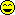Kind regards,
Mittyri
Helmut
★★★Vienna, Austria,
2015-08-15 03:22
(2558 d 11:28 ago)

@ mittyri
Posting: # 15272
Views: 10,707

## Spaghetti viennese

Hi Mittyri,

hey good idea! I merged our pasta; some cosmetics. Added a “clinical jus­ti­fi­ca­tion” flag (T|F) which forces to ABE for Cmax if FALSE:

###### input section ###### GMR      <- c(0.90, 0.90) # GMR of Cmax, AUC CV       <- c(0.55, 0.40) # CV of Cmax, AUC pwr      <- 0.8           # target power des      <- "2x2x4"       # ABEL: "2x2x4", "2x2x3", "2x3x3"                           # ABE : "2x2x2" ("2x2") just     <- TRUE          # widening for Cmax clin. justified (T|F)                           # TRUE: ABEL | FALSE: ABE ########################### ##### not implemented ##### n.min    <- 12            # minimum sample size according to GL reg      <- "EMA"         # "EMA"    (ABEL for CV >0.3)                           # "ANVISA" (ABEL for CV >0.4) ########################### library(PowerTOST) f1       <- function(x) power.TOST(CV=CV, theta0=x,                                    n=n, des=des)-pwr f2       <- function(x) power.scABEL(CV=CV, theta0=x,                                      n=n, des=des, reg=reg)-pwr f3       <- function(x) power.TOST(CV=CV, theta0=x,                                    n=n, des=des)-pwr if (des == "2x2") des <- "2x2x2" designs  <- c("2x2x2", "2x2x4", "2x2x3", "2x3x3") type     <- c("RT|TR", "RTRT|TRTR", "RTR|TRT", "RRT|RTR|TRR") trt0     <- c(2, 4, 3, 3) # treatments / subject fmt      <- paste0("%.", max(nchar(as.character(c(GMR, CV))))-2, "f") ifelse (CV <= 0.5, LL <- exp(-0.76*CV2se(CV)),   LL <- exp(-0.76*CV2se(0.5)))                    # lower scaled limit if (CV <= 0.3 | des == "2x2x2" | just == F) LL <- 0.8 # conv. limit res      <- matrix(nrow=2, ncol=10, byrow=T, dimnames=list(NULL,               c("Design", "method", "metric", "GMR", "CV",                 "LL", "UL", "n", "power", "GMRlo")))             # 1st row: method / PK-metric driving the sample size             # 2nd row: the other one + lowest GMR keeping target power res[, 1] <- type[match(des, designs)] if (des == "2x2x2" | just == FALSE) { # 2x2 or ABEL not justified   tmp.Cmax <- sampleN.TOST(CV=CV, theta0=GMR,                 targetpower=pwr, des=des, print=F) } else { # design suitable and ABEL justified   tmp.Cmax <- sampleN.scABEL(CV=CV, theta0=GMR,                 targetpower=pwr, des=des, reg=reg,                 details=F, print=F) } n.Cmax   <- tmp.Cmax[["Sample size"]] pwr.Cmax <- tmp.Cmax[["Achieved power"]] tmp.AUC  <- sampleN.TOST(CV=CV, theta0=GMR,               targetpower=pwr, des=des, print=F) # ABE n.AUC    <- tmp.AUC[["Sample size"]] pwr.AUC  <- tmp.AUC[["Achieved power"]] ifelse (n.Cmax >= n.AUC, n <- n.Cmax, n <- n.AUC) txt      <- NULL if (n.Cmax >= n.AUC) { # sample size driven by Cmax   pwr.AUC <- power.TOST(CV=CV, theta0=GMR, n=n, des=des)   GMR.AUC.lo <- uniroot(f1, interval=c(0.8, GMR), tol=1e-6)$root ifelse (CV <= 0.3 | des == "2x2x2" | just == FALSE, res[1, 2] <- "ABE", res[1, 2] <- "ABEL") if (des != "2x2x2" & just == FALSE) { txt <- paste("Expanding limits for Cmax clinically not justified", "(study will be\nevaluated by \u2013 conventional", "\u2013 ABE).\n") } txt <- paste0(txt, "The sample size is ruled by Cmax.\n", "Sample size: ", n, " (", res[1, 2], ", power: ", sprintf("%.4f", pwr.Cmax), ")\n", "Power of AUC (ABE): ", sprintf("%.4f", pwr.AUC), "\n", "Lowest GMR of AUC which will give power ", pwr, ": ", sprintf("%.4f", GMR.AUC.lo)) res[1, 3:10] <- c("Cmax", sprintf(fmt, GMR), sprintf(fmt, CV), sprintf("%.4f", LL), sprintf("%.4f", 1/LL), n, sprintf("%.4f", pwr.Cmax), NA) res[2, 2:10] <- c("ABE", "AUC", sprintf(fmt, GMR), sprintf(fmt, CV), sprintf("%.4f", 0.8), sprintf("%.4f", 1.25), n, sprintf("%.4f", pwr.AUC), sprintf("%.4f", GMR.AUC.lo)) } else { # sample size driven by AUC if (des != "2x2x2" & just == TRUE) { # ABEL if justified pwr.Cmax <- power.scABEL(CV=CV, theta0=GMR, n=n, des=des, reg=reg) GMR.Cmax.lo <- uniroot(f2, interval=c(LL, GMR), tol=1e-6)$root   } else {              # ABE     pwr.AUC <- power.TOST(CV=CV, theta0=GMR, n=n, des=des)     GMR.Cmax.lo <- uniroot(f3, interval=c(LL, GMR), tol=1e-6)\$root   }   txt <- paste0("The sample size is ruled by AUC.\n",            "Sample size: ", n, " (ABE, power: ",            sprintf("%.4f", pwr.AUC), ")\n",            "Power of Cmax")   ifelse (CV <= 0.3 | des == "2x2x2" | just == FALSE,     txt <- paste(txt, "(ABE): "),     txt <- paste(txt, "(ABEL): "))   txt <- paste0(txt, sprintf("%.4f", pwr.Cmax), "\n",            "Lowest GMR of Cmax which will give power ", pwr, ": ",            sprintf("%.4f", GMR.Cmax.lo))   res[1, 2:10] <- c("ABE", "AUC", sprintf(fmt, GMR),                     sprintf(fmt, CV), sprintf("%.4f", 0.8),                     sprintf("%.4f", 1.25), n,                     sprintf("%.4f", pwr.AUC), NA)   ifelse (CV <= 0.3, res[2, 2] <- "ABE", res[2, 2] <- "ABEL")   res[2, 3:10] <- c("Cmax", sprintf(fmt, GMR),                     sprintf(fmt, CV), sprintf("%.4f", LL),                     sprintf("%.4f", 1/LL), n,                     sprintf("%.4f", pwr.Cmax),                     sprintf("%.4f", GMR.Cmax.lo)) } txt      <- paste(txt, "\nTotal number of treatments in study:",               as.numeric(res[1, 8])*trt0[match(des, designs)], "\n") res      <- data.frame(res) print(res, row.names=F); cat(txt)

My first example:

    Design method metric  GMR   CV     LL     UL  n  power  GMRlo  RTRT|TRTR    ABE    AUC 0.90 0.40 0.8000 1.2500 68 0.8072   <NA>  RTRT|TRTR   ABEL   Cmax 0.90 0.55 0.6984 1.4319 68 0.9700 0.8435 The sample size is ruled by AUC. Sample size: 68 (ABE, power: 0.8072) Power of Cmax (ABEL): 0.9700 Lowest GMR of Cmax which will give power 0.8: 0.8435 Total number of treatments in study: 272

My second example:

    Design method metric  GMR   CV     LL     UL  n  power  GMRlo  RTRT|TRTR   ABEL   Cmax 0.90 0.35 0.7723 1.2948 34 0.8118   <NA>  RTRT|TRTR    ABE    AUC 0.95 0.25 0.8000 1.2500 34 0.9917 0.8892 The sample size is ruled by Cmax. Sample size: 34 (ABEL, power: 0.8118) Power of AUC (ABE): 0.9917 Lowest GMR of AUC which will give power 0.8: 0.8892 Total number of treatments in study: 136

And yours:

 Design method metric  GMR   CV     LL     UL  n  power  GMRlo   RT|TR    ABE   Cmax 0.95 0.29 0.8000 1.2500 38 0.8202   <NA>   RT|TR    ABE    AUC 0.94 0.25 0.8000 1.2500 38 0.8756 0.9232 The sample size is ruled by Cmax. Sample size: 38 (ABE, power: 0.8202) Power of AUC (ABE): 0.8756 Lowest GMR of AUC which will give power 0.8: 0.9232 Total number of treatments in study: 76

» Detlew, what about implementation in PowerTOST both branches?I would say that’s overkill. In PowerTOST almost everything is vec­tor­ized (i.e., you can assess different CVs for T and R, calculate power for unbalanced sequences, etc.). I wouldn’t do that.

One of the contributing authors of PowerTOST (Ben) is working on power for correlated PK-metrics. I guess that’s next we will see.

» PS: Helmut&Detlew, it seems sometimes that you're thinking on RBetter than to think in R.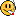To do: Force sample sizes to 12 for low CVs (according to GLs), reg <- "ANVISA" (ABEL if CV >0.4), different CVs for T and R in replicate designs, throw a warning if in a RTR|TRT-design the estimated sample size is <24.

Dif-tor heh smusma 🖖Helmut SchützThe quality of responses received is directly proportional to the quality of the question asked. 🚮
Science Quotes
d_labes
★★★

Berlin, Germany,
2015-08-17 08:45
(2556 d 06:05 ago)

@ Helmut
Posting: # 15276
Views: 10,205

## Spaghetti viennese

Dear Helmut, dear Mittyri,

» » Detlew, what about implementation in PowerTOST both branches?»
» I would say that’s overkill. In PowerTOST almost everything is vec­tor­ized (i.e., you can assess different CVs for T and R, calculate power for unbalanced sequences, etc.). I wouldn’t do that.

First: Good job.

Second: IMHO much too much coding. Why not doing simply 2 sampleN.xyz() calls and decide with own brain which metric drives the sample size? And voila - you have the first 5 results lines of the examples above.
A third function call of power.xyz() will give you the information in the sixth line of your examples (power of the metric not driving the sample size at choosen sample size).
If the last information, lowest GMR, is really needed is a matter of taste. IMHO it's only "nice to know". Educating regulators with that information would postulate that they read the sample size estimation chapter of the study protocol. I strongly doubt ...Thus, third: Full ACK with Helmut: It's overkill.

BTW: Lowest GMR which assures target power reminds me to Dave's BOSS button in FARTSSIE.

Regards,

Detlew
BE-proff
●

2015-08-17 08:55
(2556 d 05:55 ago)

@ Helmut
Posting: # 15277
Views: 10,152

Hello Helmut,

Really great job!I am taking off my hat to you...Ing. Helmut Schütz